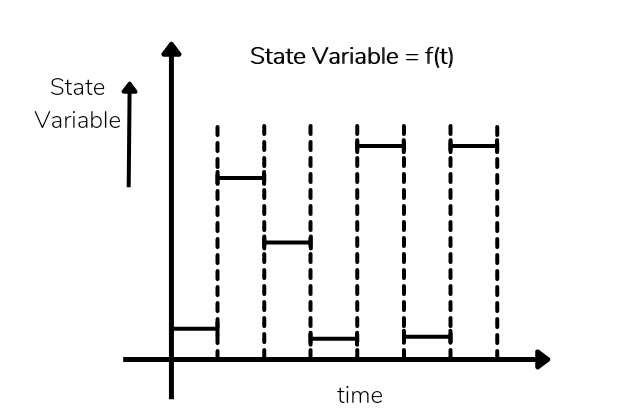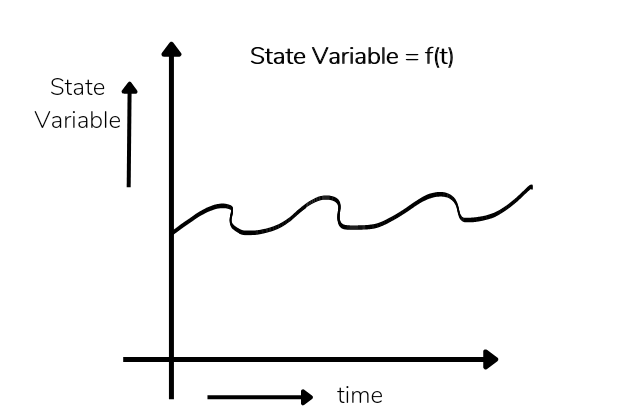# Model Classification and Types of Models in Simulation

## Model Classification

Several classification categories for model exist. Model is classified in different types , So the different kind of models are

## ✓4 Types of Models in Simulation/ Types of Simulation

i). Discrete Event Simulation Model

ii). Stochastic and Deterministic System

iii). Static and Dynamic Simulation

iv). Discrete vs Continuous System

### i). Discrete Event Simulation Model

Discrete event simulation model can be defined as one in which the state variable change only at those discrete points in time at which events occur.

Events occurs as a consequence of activity times and delays.

A discrete event simulation model is conducted overtime by a mechanism that moves simulated time forward.

The system state is updated at each event along with capturing and freezing off resources that me ok at the time.

### ii). Stochastic and Deterministic System

#### ✓Stochastic System

A system exhibits probabilistic aur stochastic behaviour if an element of change exists.

A probabilistic or stochastic model exhibits random effect.

The the behaviour cannot be predicted with certanity only probabilistic estimates can be given.

In this case the interaction between various subsystems cannot be defined with certainty.

#### ✓ Deterministic System

A system exhibits determine system it an element of change cannot exist.

A deterministic model aur system does not exhibits random effect.

The the behaviour of a deterministic system is completely known.

There is no uncertainty involved in defining the output of the system knowing the input.

The interaction between various subsystem is known with uncertainty.

### iii) Static and Dynamic Simulation

#### ✓Static System Simulation

It is the one which describe relationship that do not change with respect to time.

Static simulation are those model which having no effect of time it means output value does not depend on time.

This model do not considered time while preparing output.

Ex. Monty kar lo and steady state simulation.

#### ✓Dynamics System Simulation

It is the one which describe time varying relationship.

Dynamic simulation are those model which having effect of time in its output.

For some input there is different output in different time.

This model consider time while preparing output.

Ex. animated cartoon or a movie.

### iv) Discrete vs Continuous System

#### ✓Discrete System

In a system when state variables change only at a countable number of points in time time is called discrete model system.

Discrete system, state variable change at discrete point in time.The point in time were state variable change is called event occur or change in state.

Discrete model having finite number of states.

In discreet system time change in incremental steps.

District model is used to solve numerical method like computational procedures

Example queue length at a cash cash machine.

#### ✓Continuous System

In a system when state variables change in a continuous way and not abruptly from one state to another that is called continuous system.

In continuous system, state variables continuously as a function of time.#### ✓Continuous Model Behaviour

Continuous model having infinite number of steps. it means state variables change infinite time in continuous model.

In continuous model time change continuously.

Continuous model is used to solve ore define analytical method like deductive mathematical reasoning.

Ex. flow of air around a car.